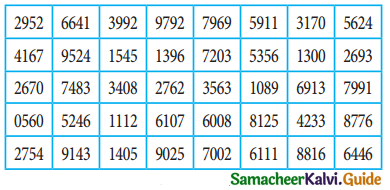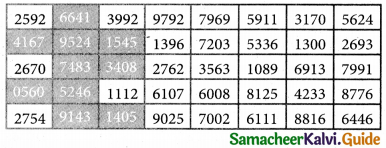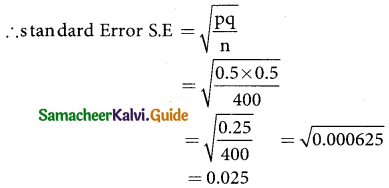Tamilnadu State Board New Syllabus Samacheer Kalvi 12th Business Maths Guide Pdf Chapter 8 Sampling Techniques and Statistical Inference Ex 8.1 Text Book Back Questions and Answers, Notes.

## Tamilnadu Samacheer Kalvi 12th Business Maths Solutions Chapter 8 Sampling Techniques and Statistical Inference Ex 8.1

Question 1.
What is population?
Solution:
The group of individuals considered under study is called as population. The word population here refers not only to people but to all items that have been chosen for the study.Question 2.
What is sample?
Solution:
A selection of a group of observation/individuals/population in such a way that is represents the population is called as sample.

Question 3.
What is statistic?
Solution:
Statistic: Any statistical measure computed from sample is known as statistic.

Question 4.
Define parameter.
Solution:
Parameter: The statistical constants of the population like mean (µ),variance (σ²) are referred as population parameters.

Question 5.
What is sampling distribution of a statistic?
Solution:
Sampling distribution of a statistic is the frequency distribution which is formed with various of a statistic computed from different samples of the same size drawn from the same population.

Question 6.
What is standard error?
Solution:
The standard deviation of the sampling distribution of a statistic is known as its Standard Error abbreviated as S.E.Question 7.
Explain in detail about simple random sampling with a suitable example.
Solution:
In this technique the samples are selected in such a way that each and every unit in the population has an equal and independent chance of being selected as a sample. Simple random sampling may be done, with or without replacement of a samples selected. In a simple random sampling with replacement there is a possibility of selecting the same sample any number of times. So, simple random sampling without replacement is followed. Thus in simple random sampling from a population of N units ,the probability of drawing any unit at the first draw is $$\frac { 1 }{N}$$, the probability of drawing any unit in the second draw from among the available (N – 1) units is $$\frac { 1 }{(N-1)}$$, and so on.

For example, if we want to select 10 students, out of 100 students, then we must write the names/roll number of all the 100 students on slips of the same size and mix them, then we make a blindfold selection of 10 students. This method is called unrestricted random sampling, because units are selected from the population without any restriction. This method is mostly used in lottery draws. If the population or universe is infinite, this method is inapplicable.

Question 8.
Explain the stratified random sampling with a suitable example.
Solution:
In stratified random sampling, first divide the population into sub-populations, which are called strata. Then,the samples are selected from each of the strata through random techniques. The collection of all the samples from all strata gives the stratified random samples.

When the population is heterogeneous or different segments or groups with respect to the variable or characteristic under study, then stratified Random sampling methos is studied. First, the population is divided into homogeneous number of sun-groups of strata before the sample is drawn. A sample from each stratum at random. Following steps are involved for selecting random sample in a stratified random sampling method.

(a) The population is divided into different classes so that each stratum will consist of more or less homogeneous elements. The strata are so designed that they do not overlap each other.

(b) After the population is stratified,a sample of a specified size is drawn at random from each stratum using Lottery Method or table of random number method.

Question 9.
Explain in detail about systematic random sampling with example.
Solution:
In a systematic sampling, randomly select the first sample from the first k units. Then every k th members, starting with the first selected sample, is included in the sample.

Systematic sampling is a commonly used technique,if the complete and up-to-date list of the sampling units is available. We can arrange the items in numerical, alphabetical, selecting the first at random, the rest being automatically selected according to some pre-determined pattern. A systematic is formed by selecting every item from the population, where k refers to the sample interval. The sampling interval can be determined by divided the size of the population by the size of the sample to be chosen.

That is K = $$\frac { N }{n}$$, where k is an integer.
k = sampling interval, size of the population, sample size
Procedure for selection of samples by systematic sampling method

(i) If we want to select a sample of 10 students from a class of 100 students,the sampling interval is Calculated as k = $$\frac { N }{n}$$ = $$\frac { 100 }{10}$$ = 10
Thus sampling interval = 10 denotes that for every 10 samples one sample

(ii) The first sample is selected from the first 10(sampling interval) samples through selection procedures.

(iii) If the selected first random sample is 5, then the rest of the samples are automatically selected by incriminating the value of the sampling interval 9k = 10. i.e, 5, 15, 25, 35, 45, 55, 65, 75, 85, 95.Question 10.
Explain in detail about sampling error.
Solution:
Sampling Error:
Error, which arise in the normal course of investigation or enumeration on account of chance, are called sampling errors, sampling errors are inherent in the method of sampling. They may arise accidentally without any bias or prejudice. Sampling Errors arise primarily due to the following reasons:
(a) Faulty selection of the sample instead of correct sample by defective sampling technique.
(b) The investigator substitutes a convenient sample if the original sample is not available while investigation.
(c) In area surveys,while dealing with border lines it depends upon the investigator whether to include them in the sample or not. This is known as faulty demarcation of sampling units.

Question 11.
Explain in detail about non-sampling error.
Solution:
Non-sampling Errors:
The errors that arise due to human factors which always vary from one investigator to another in selecting, estimating or using measuring instruments (tape, scale) are called Non¬sampling errors. It may arise in the following ways:
(a) Due to neglience and carelessness of the part of either investigator or respondents
(b) Due to lack of trained and qualified investigators.
(c) Due to framing of a wrong questionnaire.
(d) Due to apply wrong statistical measure.
(e) Due to incomplete investigation and sample survey.

Question 12.
State any two merits of simple random sampling.
Solution:
Merits:
1. Personal bias is completely eliminated.
2. This method is economical as it saves time, money and labour.

Question 13.
State any three merits of stratified random sampling.
Solution:
Merits:
(a) A random stratified sample is superior to a sample random sample because it ensures representation of all groups and thus it is more representative of the population which is being sampled.
(b) A stratified random sample can be kept small in size without losing its accuracy
(c) it is easy to administer, if the population under study is sub divided
(d) It reduces the time and expenses in dividing the strata into geographical divisions, since the government itself had the geographical areas.

Question 14.
State any two demerits of systematic random sampling.
Solution:
Demerits:
1. Systematic samples are not random samples.
2. If N is not multiple of n-then the sampling interval (k) cannot be an integer, thus sample selection becomes difficult.

Question 15.
State any two merits for systematic random sampling.
Solution:
Merits:
1. This is simple and convenient method.
2. This method distributes the sample more evenly over the entire listed population.
3. The time and work is reduced much.Question 16.
Using the following Tippet’s random number tableDraw a sample of 10 three digit numbers which are even numbers.
Solution:
There are many ways to select 10 random samples from the given Tippets random number number table since the population size is three digit numbers, Here the door numbers must be even (ie) the unit digit must be even. Here we consider column wise selection of random numbers starting from first column.

So the first sample is 416 and other 9 samples are 056, 664, 952, 748, 524, 914, 154, 340 and 140.
Tippets random number TableQuestion 17.
A wholesaler in apples claims that only 4% of the apples supplied by him are defective. A random sample of 600 apples contained 36 defective apples. Calculate the standard error concerning of good apples.
Solution:
sample size = 600; Number of success = 600 – 36
= 564
sample proportion p = $$\frac { 564 }{600}$$ = 0.94
600
population proportion (p) = probability of getting good apple
= 96%
= $$\frac { 96 }{100}$$ {∵ 4% of the apples 100 are defective}
P = 0.96
Q = 1 – p = 1 – 0.96
Q = 0.04
The S.E for a sample proporation is given by
S.E = $$\sqrt{\frac { PQ }{N}}$$ = $$\sqrt{\frac { (0.96)(0.04) }{600}}$$
$$\sqrt{\frac { 0.0384 }{600}}$$ = $$\sqrt{0.000064}$$
∴ S.E = 0.008
Hence the standard error foe sample proportion is S.E = 0.008Question 18.
A sample of 1000 students whose mean weight is 119 lbs (pounds) from a school in Tamil Nadu State was taken and their average weight was found to be 120 lbs with a standard deviation of 30 lbs. Calculate standard error of mean.
Solution:
Given n = 1000; $$\bar{x}$$ = 119 lbs (pounds)
s = 30 lbs is known in this problem.
since σ is unknown, so we consider $$\bar{σ}$$ = s and µ = 120 lbs
S.E = $$\frac { \bar{σ} }{√n}$$ = $$\frac { s }{√n}$$ = $$\frac { 30 }{\sqrt{1000}}$$
= $$\frac { 30 }{31.623}$$ = 0.9487
Therefore the standard error for the average weight of large group of students of 120 lbs is 0.9487

Question 19.
A random sample of 60 observations was drawn from a large population and its standard deviation was found to be 2.5. Calculate the suitable standard error that this sample is taken from a population with standard deviation 3?
Solution:
Sample size n = 60
Sample S.D S = 2.5
population S.D a = 3
The standard error for sample S.D is given by
$$\sqrt{\frac { σ^2 }{2n}}$$ = $$\sqrt{\frac { (3)^2 }{2(60)}}$$ = $$\frac { 3 }{\sqrt{120}}$$
= $$\frac { 3 }{10.954}$$ = 0.27387
= 0.2739
Thus standard error for sample S.D = 0.2739

Question 20.
In a sample of 400 population from a village 230 are found to be eaters of vegetarian items and the rest non-vegetarian items. Compute the standard error assuming that both vegetarian and non-vegetarian foods are equally popular in that village?
Solution:
sample size n = 400
case (i):
sample proporation of vegetarian p = $$\frac { 3 }{10.954}$$ = $$\frac { 230 }{400}$$
p = 0.575
q = 1 – p
= 1 – 0.575
q = 0.425
Sample error S.E= $$\sqrt{\frac { pq }{n}}$$
= $$\sqrt{\frac { 0.575×0.425 }{400}}$$ = $$\sqrt{\frac { 0.223125 }{400}}$$
$$\sqrt{0.0005578125}$$
S.E = 0.2361

Case(ii):
sample size n = 400
since both vegetarian and non- vegetarian foods are equally popular in that village
sample proparation of vegetarian p = $$\frac { 1 }{2}$$ = 0.5
q = 1 -p ⇒ q = 1 – 0.5
q = 0.5